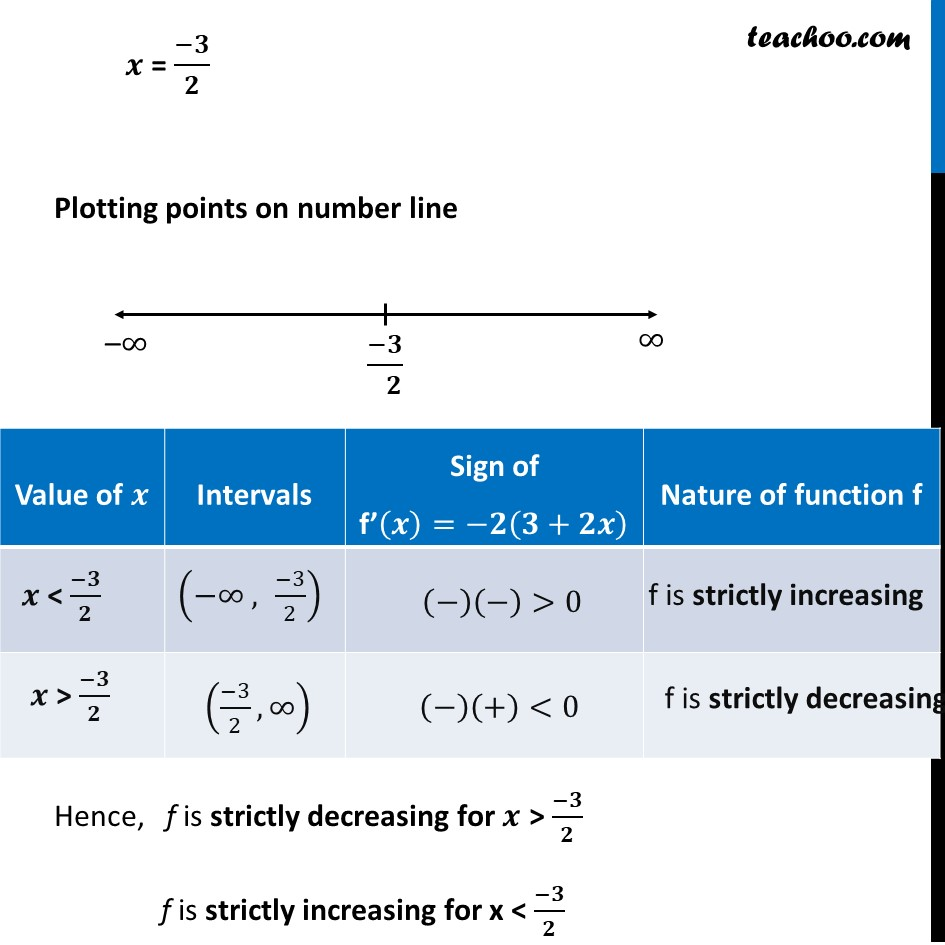Ex 6.2

Chapter 6 Class 12 Application of Derivatives
Serial order wiseLearn in your speed, with individual attention - Teachoo Maths 1-on-1 Class

### Transcript

Ex 6.2, 6 Find the intervals in which the following functions are strictly increasing or decreasing: (b) 10 – 6𝑥 – 2𝑥2 Calculating f’(𝒙) f(𝑥) = 10 – 6𝑥 – 2𝑥2 f’(𝑥) = –6 – 4𝑥 f’(𝑥) = −2(3 + 2𝑥) Putting f’(𝒙) = 0 −2(3 + 2𝑥) = 0 3 + 2𝑥 = 0 2𝑥 = −3 𝒙 = (−𝟑)/𝟐 Plotting points on number line Hence, f is strictly decreasing for 𝒙 > (−𝟑)/𝟐 f is strictly increasing for x < (−𝟑)/𝟐